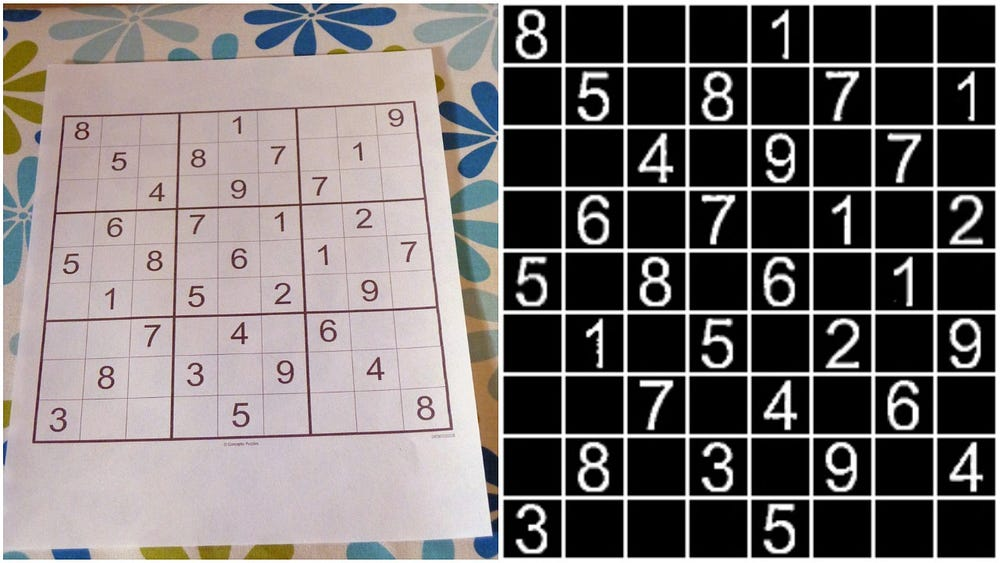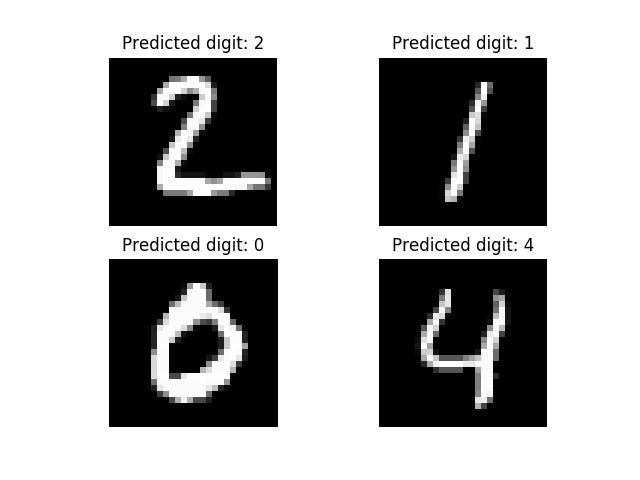Sudoku Solver using OpenCV and DL — Part 2

Source: Deep Learning on Medium

If you’re impatient, scroll to the bottom of the post for the Github Repos

This is Part 2 of Sudoku Solver. Make sure you got a glimpse of Part 1. So moving ahead, till now we have preprocessed an image i.e., take an image and perform a crop and warp perspective transform. Now we need to extract the numbers and solve the sudoku.Original Sudoku image and pre performed image

B: Extract each number present in the image

So, our next task is to extract each number from the image, identify the number and save it into a 2D matrix.

For digit recognition, we will be training neural network over MNIST dataset containing 60,000 images of digits from 0 to 9.

We will start by importing all the libraries.

import numpy
import cv2from keras.datasets
import mnistfrom keras.models
import Sequentialfrom keras.layers
import Densefrom keras.layers
import Dropoutfrom keras.layers
import Flattenfrom keras.layers.convolutional
import Conv2Dfrom keras.layers.convolutional
import MaxPooling2Dfrom keras.utils
import np_utilsfrom keras
import backend as K
import matplotlib.pyplot as plt

We will fix random seed for reproducibility.

K.set_image_dim_ordering('th')
seed = 7numpy.random.seed(seed)
(X_train, y_train), (X_test, y_test) = mnist.load_data()

Now, let’s reshape the images to be samples*pixels*width*height and normalize inputs from 0–255 to 0–1. After this, we will one hot encode the outputs.

X_train = X_train.reshape(X_train.shape, 1, 28,
28).astype('float32')
X_test = X_test.reshape(X_test.shape, 1, 28,
28).astype('float32')
X_train = X_train / 255
X_test = X_test / 255
y_train = np_utils.to_categorical(y_train)
y_test = np_utils.to_categorical(y_test)
num_classes = y_test.shape

Next, we will create a model to predict the handwritten digit.

model = Sequential()
model.add(Conv2D(32, (5, 5), input_shape=(1, 28, 28),
activation='relu'))
3), activation='relu'))

After creating the model, let’s compile it and fit over the dataset and evaluate it.

metrics=['accuracy'])
model.fit(X_train, y_train, validation_data=(X_test, y_test),
epochs=10, batch_size=200)
scores = model.evaluate(X_test, y_test, verbose=0)
print("Large CNN Error: %.2f%%" % (100-scores*100))

Now, we will test the above model created.

test_images = X_test[1:5]
test_images = test_images.reshape(test_images.shape, 28, 28)
print ("Test images shape: {}".format(test_images.shape))
for i, test_image in enumerate(test_images, start=1):
org_image = test_image
test_image = test_image.reshape(1,1,28,28)
prediction = model.predict_classes(test_image, verbose=0)
print ("Predicted digit: {}".format(prediction))
plt.subplot(220+i)
plt.axis('off')
plt.title("Predicted digit: {}".format(prediction))
plt.imshow(org_image, cmap=plt.get_cmap('gray'))
plt.show()Predicted digits of Handwritten digit classification model

The accuracy of the neural network was 98.314%. At last, we will save the sequential model so that we don’t have to train it over and over whenever we want to use it.

# serialize model to JSON
model_json = model.to_json()
with open("model.json", "w") as json_file:
json_file.write(model_json)
# serialize weights to HDF5
model.save_weights("model.h5")
print("Saved model to disk")

To know more about Handwritten Digit Recognition https://github.com/aakashjhawar/Handwritten-Digit-Recognition

Our next step will be to load the pre-trained model and weights.

json_file = open('model.json', 'r')
json_file.close()

Then we will resize the image and split the image into 9×9 small images. Each small image will be of a digit from 1- 9.

sudoku = cv2.resize(sudoku, (450,450))
grid = np.zeros([9,9])
for i in range(9):
for j in range(9):
image = sudoku[i*50:(i+1)*50,j*50:(j+1)*50]
if image.sum() > 25000:
grid[i][j] = identify_number(image)
else:
grid[i][j] = 0
grid = grid.astype(int)

identify_number function takes an image of digit and predicts the digit in the image.

def identify_number(image):
image_resize = cv2.resize(image, (28,28)) # For plt.imshow
image_resize_2 = image_resize.reshape(1,1,28,28) # For input to model.predict_classes
# cv2.imshow('number', image_test_1)
, verbose = 0)

After performing the above steps our Sudoku grid will look like:

C: Compute the solution of the sudoku using Backtracking Algorithm

We will use Backtracking Algorithm to compute the solution of the sudoku.

Search the grid to find an entry that is still unassigned. If found, the reference parameters row, col will be set the location that is unassigned, and true is returned. If no unassigned entries remain, false is returned. ‘l’ is a list variable that has been passed from the solve_sudoku function to keep track of incrementation of rows and columns.

def find_empty_location(arr,l):
for row in range(9):
for col in range(9):
if(arr[row][col]==0):
l=row
l=col
return True
return False

Returns a boolean which indicates whether any assigned entry in the specified row matches the given number.

def used_in_row(arr,row,num):
for i in range(9):
if(arr[row][i] == num):
return True
return False

Returns a boolean which indicates whether any assigned entry in the specified column matches the given number.

def used_in_col(arr,col,num):
for i in range(9):
if(arr[i][col] == num):
return True
return False

Returns a boolean which indicates whether any assigned entry within the specified 3×3 box matches the given number.

def used_in_box(arr,row,col,num):
for i in range(3):
for j in range(3):
if(arr[i+row][j+col] == num):
return True
return False

Checks whether it will be legal to assign num to the given (row, col). Returns a boolean which indicates whether it will be legal to assign num to the given (row, col) location. Check if ‘num’ is not already placed in the current row, current column and current 3×3 box.

def check_location_is_safe(arr,row,col,num):
return not used_in_row(arr,row,num) and
not used_in_col(arr,col,num) and
not used_in_box(arr,row - row%3,col - col%3,num)

Takes a partially filled-in grid and attempts to assign values to all unassigned locations in such a way to meet the requirements for Sudoku solution (non-duplication across rows, columns, and boxes). ‘l’ is a list variable that keeps the record of row and col in find_empty_location function. Assigning list values to row and col that we got from the above function.

def solve_sudoku(arr):
l=[0,0]
if(not find_empty_location(arr,l)):
return True
row=l
col=l
for num in range(1,10):
if(check_location_is_safe(arr,row,col,num)):
arr[row][col]=num
if(solve_sudoku(arr)):
return True
# failure, unmake & try again
arr[row][col] = 0

return False

The last thing is to print the grid.

def print_grid(arr):
for i in range(9):
for j in range(9):
print (arr[i][j])
print ('\n')

Now, let’s stitch all the functions in the main function.

def sudoku_solver(grid):
if(solve_sudoku(grid)):
print('---')
else:
print ("No solution exists")
grid = grid.astype(int)
return grid

The output of this function will be our solved sudoku.